### Control Systems in Python - Part 2 - Routh Hurwitz

In my last post Control Systems in Python Part 1, i described how to setup and use Python for doing some basic plotting of transfer functions. One of the biggest benefits of using sympy vs numeric packages like matlab/numpy/scipy is the fact that you can use symbolic variables. This post includes a function for computing the Routh Hurwitz table (Note: It does not work for row's of zeros).

Lets do my control systems design homework problem together :) (Warning: I have not verified if this answer is right so please correct me if it’s not!)

The Problem

The problem is DP 9.11 from Dorf & Bishop’s Modern Control Systems. ISBN 0136024580. Basically we have to design a controller to compensate for a system with a time delay.
The controller is: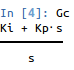And the system is: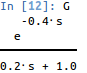First we approximate the exponential term with a 2nd order polynomial using pade(0.4,2) such that: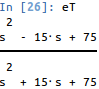Thus the approximated system is: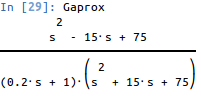Using frequency response methods, design the controller so that the overshoot of the system is P.O. <= 10%. From this we determine 0.59. When we plot the poles and zeros of the system we see that there are 3 poles in the LHP and two zeros in the RHP. The controller adds another pole at 0, so the zero of our controller should be somewhere to the left of the -5 pole on the real axis.
Thus Ki/Kp > 5. If Ki/Kp = 6. Using a Routh Hurwitz table we can see that 0<Kp<0.66 makes the system stable.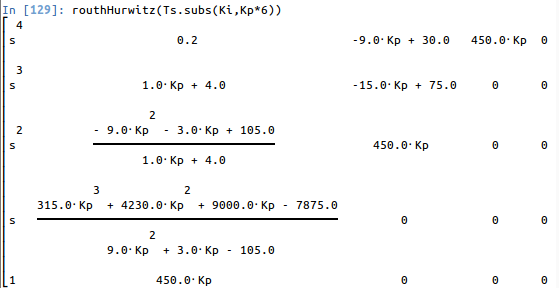The code for this function is:

def routhHurwitz(Ts,*args):
den = Poly(Ts.as_numer_denom(),s).all_coeffs()
n = len(den)-1
if n < 2:
return None
m = matrices.zeros(n+1)
# Insert the first two rows
c = [den[i] for i in range(0,n+1,2)]
for i in range(0,len(c)):
m[0,i] = c[i]
c = [den[i] for i in range(1,n+1,2)]
for i in range(0,len(c)):
m[1,i] = c[i]

# Compute the rest of the entries
for i in range(2,n+1):
for j in range(1,n):
if m[i-1,j] == 0:
m[i,j-1] = m[i-2,j]
elif m[i-1,0] !=0:
m[i,j-1] = ((m[i-1,0]*m[i-2,j]-m[i-2,0]*m[i-1,j])/m[i-1,0]).together().simplify()

m = m.col_insert(0, Matrix(([s**(n-i) for i in range(0,n+1)])))
return m

When we set Kp = 0.22 we get the controller: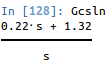giving the step response of: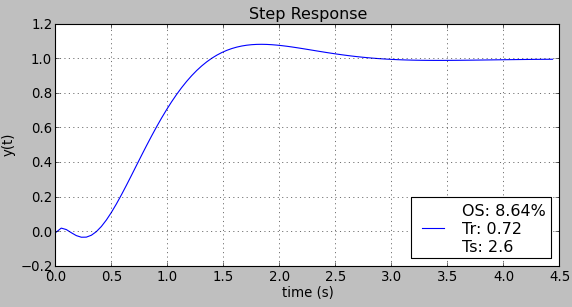if the gain is reduced the %OS reduces and Ts rises.